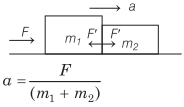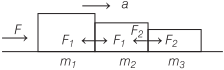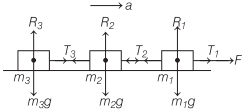# Motion of Bodies in Contact | Definition, Types – Laws of Motion

## Motion of Bodies in Contact | Definition, Types – Laws of Motion

We are giving a detailed and clear sheet on all Physics Notes that are very useful to understand the Basic Physics Concepts.

Motion of Bodies in Contact
1. Contact Force Between Two Blocks:
If force F is applied on an object of mass m1, then acceleration of the bodiesContact force on m1 = m1a = $$\frac{m_{1} F}{\left(m_{1}+m_{2}\right)}$$
Contact force on m2 = m2a = $$\frac{m_{2} F}{\left(m_{1}+m_{2}\right)}$$

2. Contact Force Between Three Blocks:
If force F is applied on an object of mass m1, then acceleration of the bodies
= $$\frac{F}{\left(m_{1}+m_{2}+m_{3}\right)}$$Contact force between m1 and m2
$$F_{1}=\frac{\left(m_{2}+m_{3}\right) F}{\left(m_{1}+m_{2}+m_{3}\right)}$$
Contact force between m2 and m3
$$F_{2}=\frac{m_{3} F}{\left(m_{1}+m_{2}+m_{3}\right)}$$

3. Motion of Two Bodies One Resting on the Other:
(a) The coefficient of friction between surface of A and B be μ. If a force F is applied on the lower body A, then common acceleration of two bodiesPseudo force acting on block B due to the accelerated motion,
f’ = ma
The pseudo force tends to produce a relative motion between bodies A and B and consequently a frictional force
f = μ N = μmg is developed.
For equilibrium, ma ≤ μmg or a ≤ μg

(b) Let friction is also present between the ground surface and body A Let the coefficient of friction between the given surface and body A is μ1 and the coefficient of friction between the surfaces of bodies A and B is μ2. If a force F is applied on the lower body A.Net accelerating force F – fA = F – μ1 (M + m)g
∴ Net acceleration
$$a=\frac{F-\mu_{1}(M+m) g}{(M+m)}=\frac{F}{(M+m)}-\mu_{1} g$$

Pseudo force acting on the block B,
f’ = ma
The pseudo force tends to produce a relative motion between the bodies A and B are consequently a frictional force fB = μ2 mg is developed. For equilibrium
ma ≤ μ2mg or a ≤ μ2g
If acceleration produced under the the effect of force Fis more than μ2g, then two bodies will not move together.

4. Motion of Bodies Connected by a String:Acceleration of the system a = $$\frac{F}{\left(m_{1}+m_{2}+m_{3}\right)}$$
Tension in string
T1 = F
T2 = (m2 + m3) a = $$\frac{\left(m_{2}+m_{3}\right) F}{\left(m_{1}+m_{2}+m_{3}\right)}$$
T3 = m3a = $$\frac{m_{3} F}{\left(m_{1}+m_{2}+m_{3}\right)}$$

Laws of Motion:
There are various laws in Physics that define the motion of the object. When an object is in motion whether it is linear or circular there is some force which is always imposed on it.